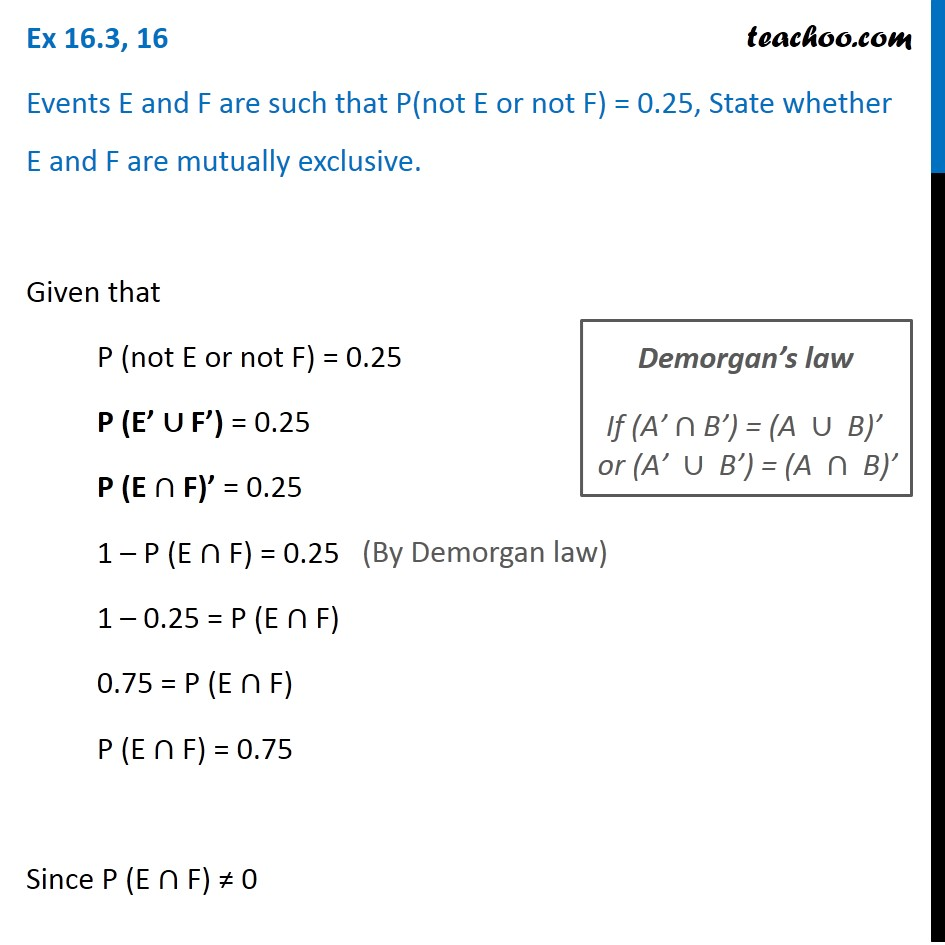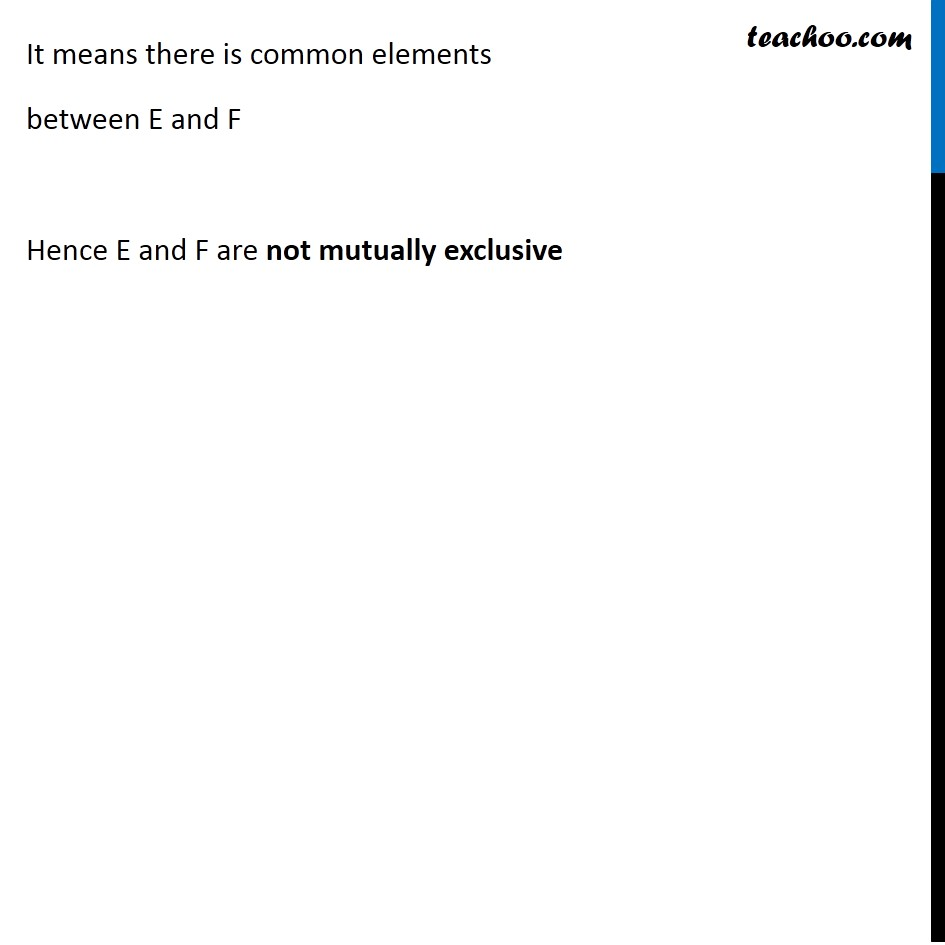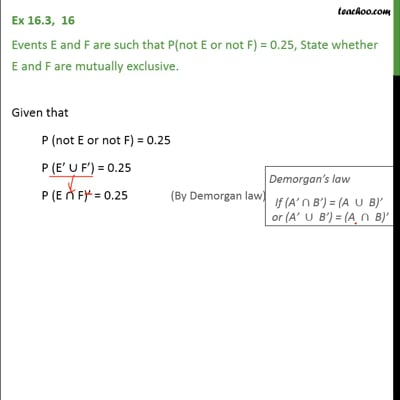Ex 16.3

Chapter 16 Class 11 Probability
Serial order wiseThis video is only available for Teachoo black users

Solve all your doubts with Teachoo Black (new monthly pack available now!)

### Transcript

Ex 16.3, 16 Events E and F are such that P(not E or not F) = 0.25, State whether E and F are mutually exclusive. Given that P (not E or not F) = 0.25 P (E’ ∪ F’) = 0.25 P (E ∩ F)’ = 0.25 1 – P (E ∩ F) = 0.25 1 – 0.25 = P (E ∩ F) 0.75 = P (E ∩ F) P (E ∩ F) = 0.75 Since P (E ∩ F) ≠ 0 (By Demorgan law) Demorgan’s law █("If (A’" ∩"B’) = (A " ∪" B)’ " @"or (A’ " ∪" B’) = (A " ∩" B)’" ) It means there is common elements between E and F Hence E and F are not mutually exclusive